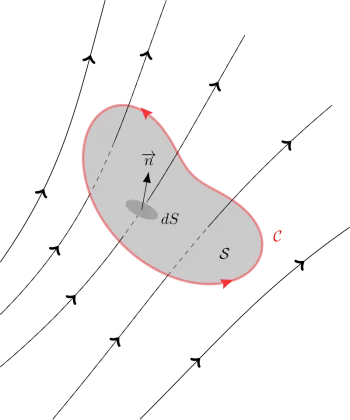# State and Explain Faraday's Law of Electromagnetic Induction and ExamplesFaraday's law of electromagnetic induction states that the induced voltage in a closed circuit is directly proportional to the speed with which the magnetic flux that crosses any surface with the circuit as an edge varies.

In other words, it states that the electromotive force induced in a conducting circuit is proportional to the rate of change of the magnetic flux link within the circuit.

The law of electromagnetic induction is the basic law of electromagnetism that helps predict how a magnetic field would interact with an electrical circuit to produce an electromotive force (emf). It describes the magnitude of the electromotive force or voltage induced in a conductor due to electromagnetic induction.

Faraday's law describes the magnitude of the electromotive force or voltage induced in a conductor due to electromagnetic induction. It states that the electromotive force induced in a conducting circuit is proportional to the rate of change of the magnetic flux link within the circuit.

The phenomenon of mutual induction occurs when two or more coils are magnetically linked to each other, and an AC flows through one loop, causing an induced emf across the other linked coils.

The law is named after the physicist and chemist who developed it, Michael Faraday, in 1831 due to his studies developed in the previous years.

In his development, Michael Faraday was based on the principle of conservation of energy. Faraday hypothesized that if the flow of an electric current could generate a magnetic field, it would also be possible that the magnetic field could produce an electric current.

## Faraday's Law of Induction Formula

The following formula defines the relationship between the variation of the magnetic flux that passes through a surface S, which is closed by the contour C, and the electric field along the same contour:where

• E represents the electric field.

• B is the magnetic flux density.

• dl is an infinitesimal element of contour C.

• dA is the differential element of the surface S.

With the right-hand rule, we can know the directions of the contours C and of dA.

The absolute value of the circulation of the electric field is equal to the rate of change of the magnetic flux through the area enclosed. The flux linkage of the coil is the product of the flux associated with the coil and the number of turns in the coil.

In the case of an inductor coil with N number of turns of the electric wire, it makes N turns; we have the following formula:where

• ε is the induced electromotive force (emf)

• dΦ / dt is the rate of change over time of the magnetic flux Φ.

Lenz's law introduced the negative sign of the formula and the direction of the electromotive force.

Faraday's law of induction was the last law to be added to Maxwell's equations.

## Examples of Applications of Faraday's Law in Daily Life

Here are some examples of the application of Faraday's law.

### 1. Electric Generator

Electric generators convert rotational kinetic energy into electricity by rotating a magnet called a rotor. The rotor rotates around fixed coils generating a changing magnetic field that induces an electric current.

Depending on the configuration, the current generator can be direct current, alternating current, or three phases.

### 2. Electric Motor

The electric motor works in a reverse way to the generator. In an electric motor, a current is applied to an electromagnet that generates a magnetic field. This field interacts with the rotor magnet causing it to rotate.

### 3. Magnetic Brake

A magnetic brake works by connecting an electromagnet to a metal disc.

To activate the brake, we generate a current through the electromagnet to generate a different polarity of a coil of wires. The electric current generates a magnetic field on the disk. The magnetic field induces the so-called eddy currents by Faraday's law.

Eddy currents are affected by the Joule effect and release heat, which is energy that comes from the kinetic energy of the disk. By reducing kinetic energy, the puck slows down.

### 4. Induction Cookers

An induction cooker also works by Joule's law generating eddy currents. An electromagnet made of several turns in a coil is placed under the ceramic glass plate. Placing a metal container on the plate activates the magnet, inducing eddy currents and, therefore, heat.

### 5. Lights Adapted to Bicycles

This kind of light found on bikes turns on when the wheel rotates thanks to a magnet attached to it that, when rotating, an EMF is induced and creates a magnetic field, which in turn produces a small energy source.

This electrical charge is transferred to the bulb and is what generates the light.

### 6. Doorbells

The process carried out by these devices involves the movement of an electrical charge through an electromagnet created using a loop of wire. The generated magnetic field strength makes an attraction that causes the metal hammer to strike a bell, stopping the circuit and restarting the process.

It allows the hammer to hit it repeatedly and produce a sound.

Author:

Published: October 16, 2021
Last review: October 16, 2021